# Test Equipment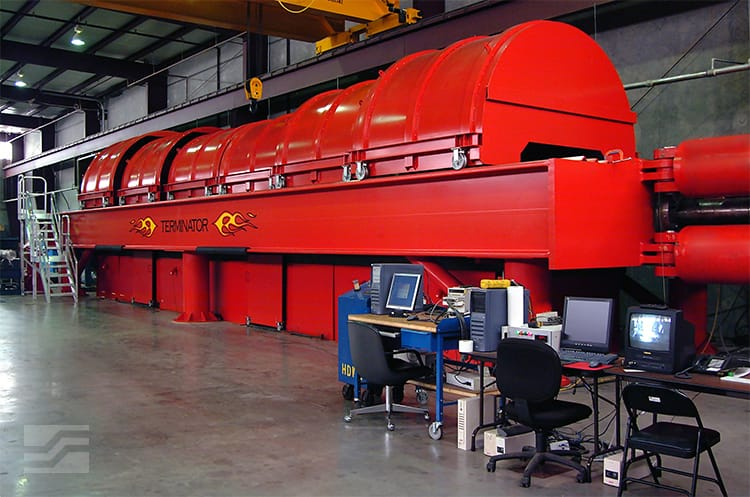### 6 Million Pound Load Frame

• Max Tension Capacity = 6,000,000 lbs
• Max Compression Capacity = 4,700,000 lbs
• Max Bending Capacity = 1,000,000 ft-lbs
• Max Stroke = 72 inches
• Max Sample Length = 44 feet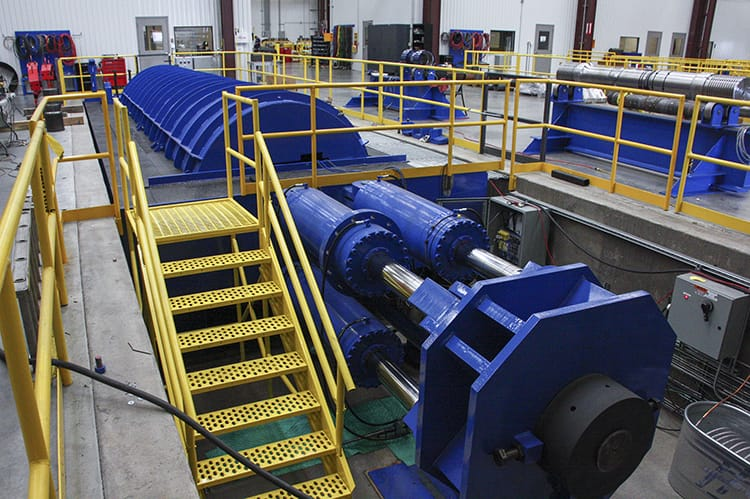### 4 Million Pound Load Frame

• Max Tension Capacity = 4,000,000 lbs
• Max Compression Capacity = 3,200,000 lbs
• Max Bending Capacity = 500,000 ft-lbs
• Max Stroke = 32 inches
• Max Sample Length = 27 feet
• N2 Pressure = 45 ksi
• H2O Pressure = 60 ksi
• Induction Heating to HPHT Conditions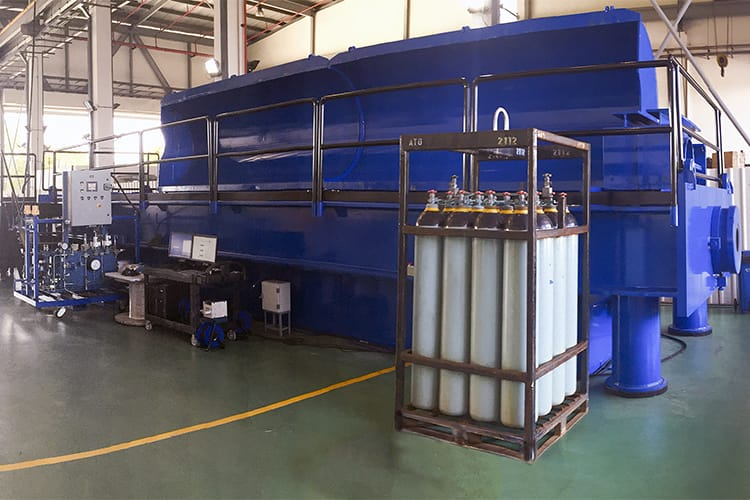• Max Tension Capacity = 3,500 kips (15.6 MN)
• Max Compression Capacity = 3,500 kips (15.6 MN)
• Max Bending Capacity = 400,000 ft-lbs (677.9 KN-m)
• Max Stroke = 36 in (91.4 cm)
• Max Sample Length = 30 ft (9.1 m)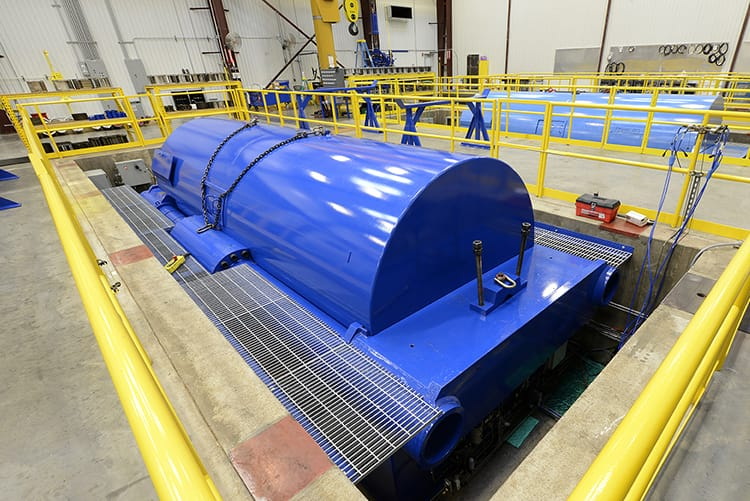### 3.3 Million Pound Load Frame

• Max Tension Capacity = 3,300,000 lbs
• Max Compression Capacity = 3,000,000 lbs
• Max Bending Capacity = 400,000 ft-lbs
• Max Stroke = 36 inches
• Max Sample Length = 11 feet
• N2 Pressure = 45 ksi
• H2O Pressure = 60 ksi
• Induction heating to HPHT Conditions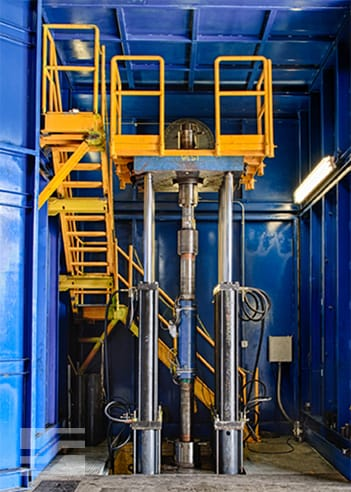### 3 Million Pound Load Frame

• Maximum Load Capacity with 60” Travel
• Tension = 3,000,000 lbs
• Compression = 2,250,000 lbs
• Data Acquisition System with up to 384 Channels
• Frame Calibration Traceable to the NIST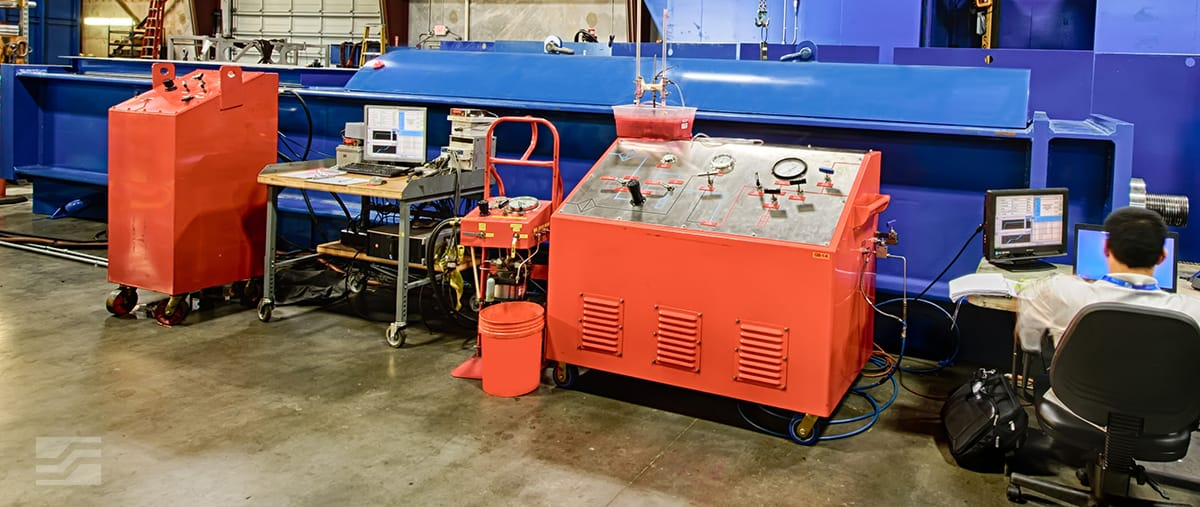### 2.5 Million Pound Load Frame

• Max Tension Capacity = 2,500,000 lbs
• Max Compression Capacity = 2,500,000 lbs
• Max Stroke = 24 inches
• Max Sample Length = 180 inches
• N2 Pressure to 45 ksi
• H20 Pressure to 60 ksi
• Induction Heating to HPHT Conditions2 Million Pound Vertical Test Frame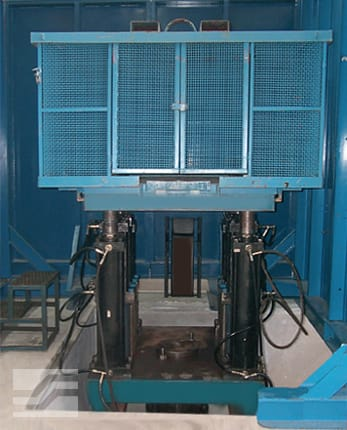### 2 Million Pound Vertical Test Frame

• Maximum Load Capacity with 60” Travel
• Tension = 2,000,000 lbs
• Compression = 1,500,000 lbs
• Data Acquisition System with up to 384 Channels
• Frame Calibration Traceable to the NIST2 Million Pound Test Frame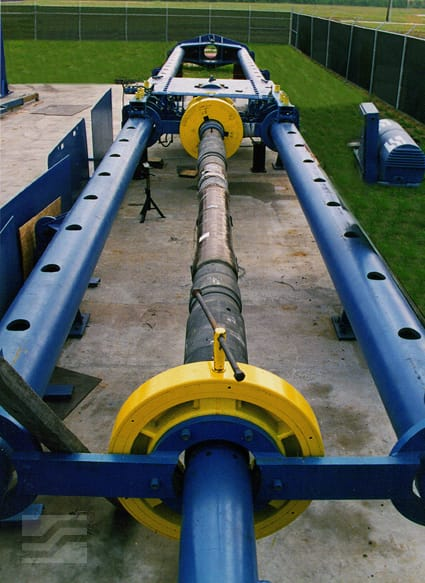### 2 Million Pound Test Frame

• Max Tension Capacity = 2,000,000 lbs
• Max Compression Capacity = 2,000,000 lbs
• Max Bending Capacity = 120,000 ft-lbs
• Max Stroke = 60 inches
• Max Sample Length = 50 feet1.0 Million Pound Test Frame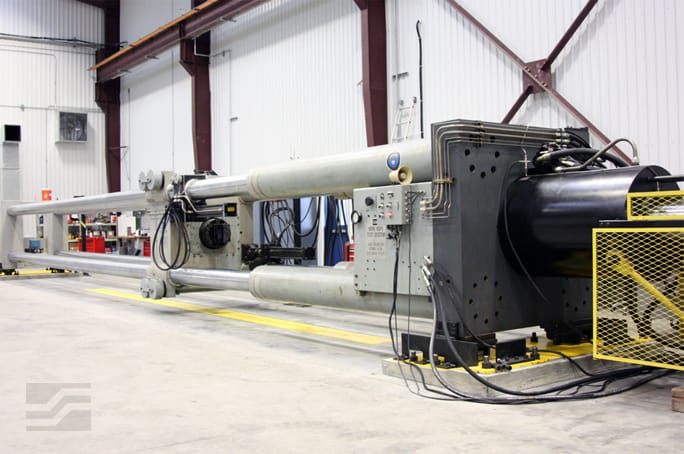### 1.0 Million Pound Test Frame

• Max Tension Capacity = 1,000,000 lbs
• Max Compression Capacity = 1,000,000 lbs
• Max Bending Capacity = 200,000 ft-lbs
• Max Stroke = 30 inches
• Max Sample Length = 45 feetMooring Rope Fatigue Frame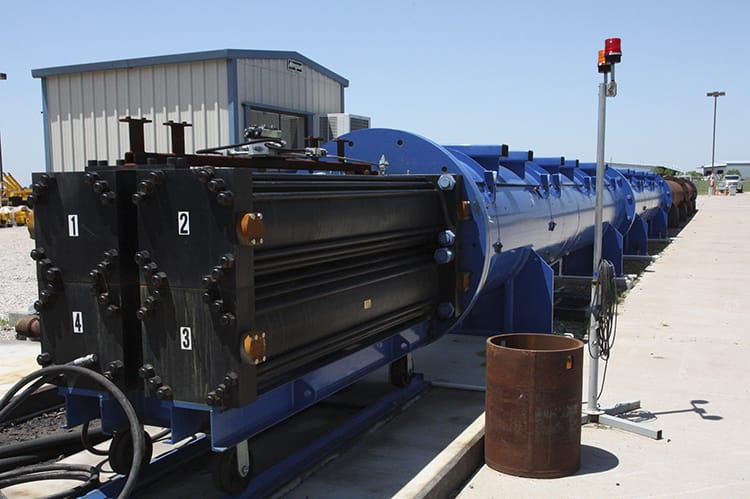### Mooring Rope Fatigue Frame

• Max Stroke = 78 feet
• Max Sample Length = 115 feet
• Designed to Test Four Sub-Ropes in Parallel1 Million Pound Test Frame - D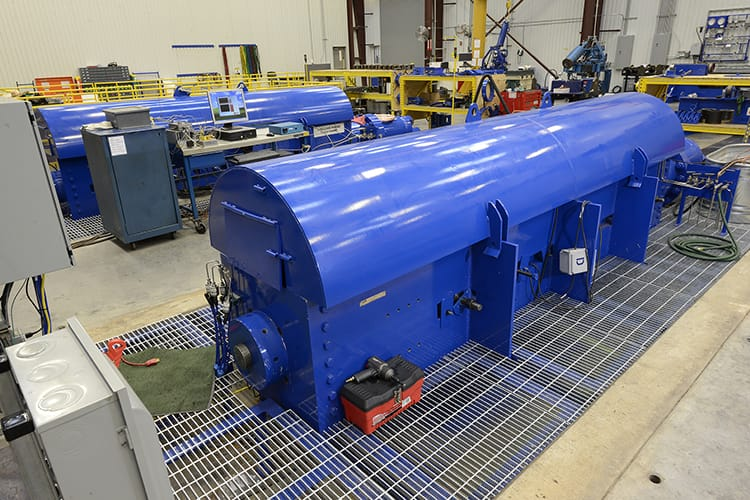### 1 Million Pound Test Frame - D

• Max Tension Capacity = 1,000,000 lbs
• Max Compression Capacity = 1,000,000 lbs
• Max Bending Capacity = 120,000 ft-lbs
• Max Stroke = 10 feet
• Max Sample Length = 22 inches
• H20 Pressure to 60 ksi
• Induction Heating to HPHT Conditions
• N2 Pressure to 45 ksi

## Torque150 ft-kip Makeup/Breakout Station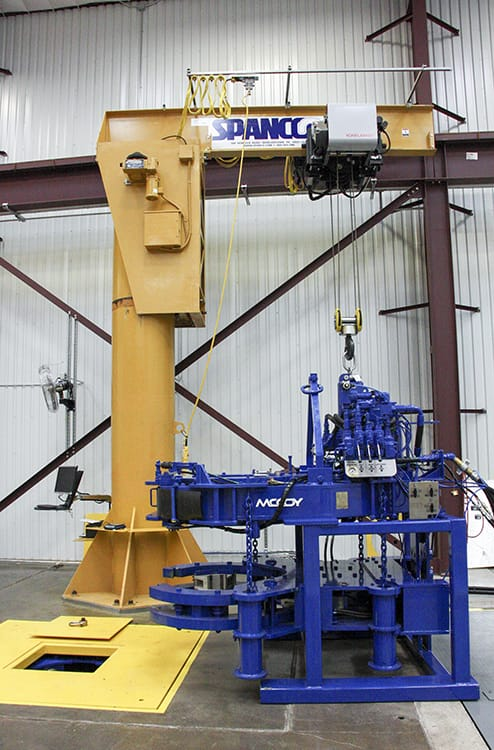### 150 ft-kip Makeup/Breakout Station

• Computerized Torque vs. Turns Control System
• Torque vs. Turns Data Recorded and Plotted
• Hydraulic Tongs to Fit Pipe Sizes from 10.75” to 22”
• Torque Capacity to 150,000 ft-lbs
• 7.5 Ton Jib Crane
• 25 Ton Bridge Crane
• One Floor Hole – 24” diameter x 20 ft deep
• In-floor Hydraulic Back-up90 & 85 ft-kip Makeup/Breakout Station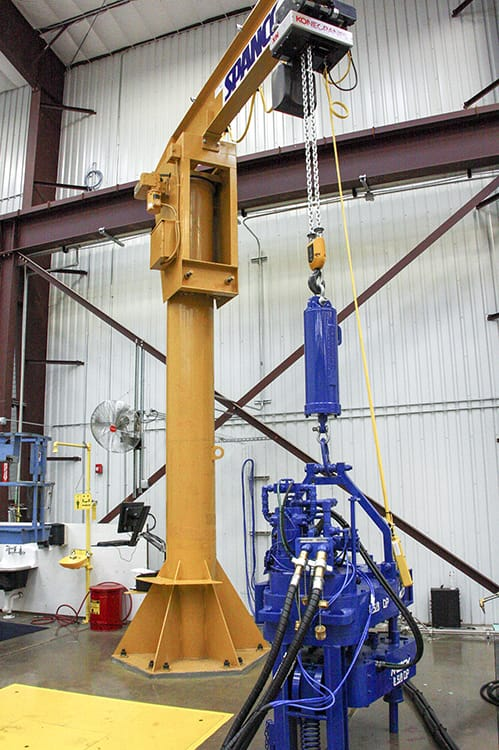### 90 & 85 ft-kip Makeup/Breakout Station

• Computerized Torque vs. Turns Control System
• Torque vs. Turns Data Recorded and Plotted
• Hydraulic Tongs to Fit Pipe Sizes:
• 7” to 14” (90,000 ft lb)
• 2.375” to 8.625” (85,000 ft lb)
• 5 Ton Jib Crane
• 10 Ton Bridge Crane
• One Floor Hole – 24” diameter x 20 ft deep
• In-floor Hydraulic Back-up

## MaterialsMaterials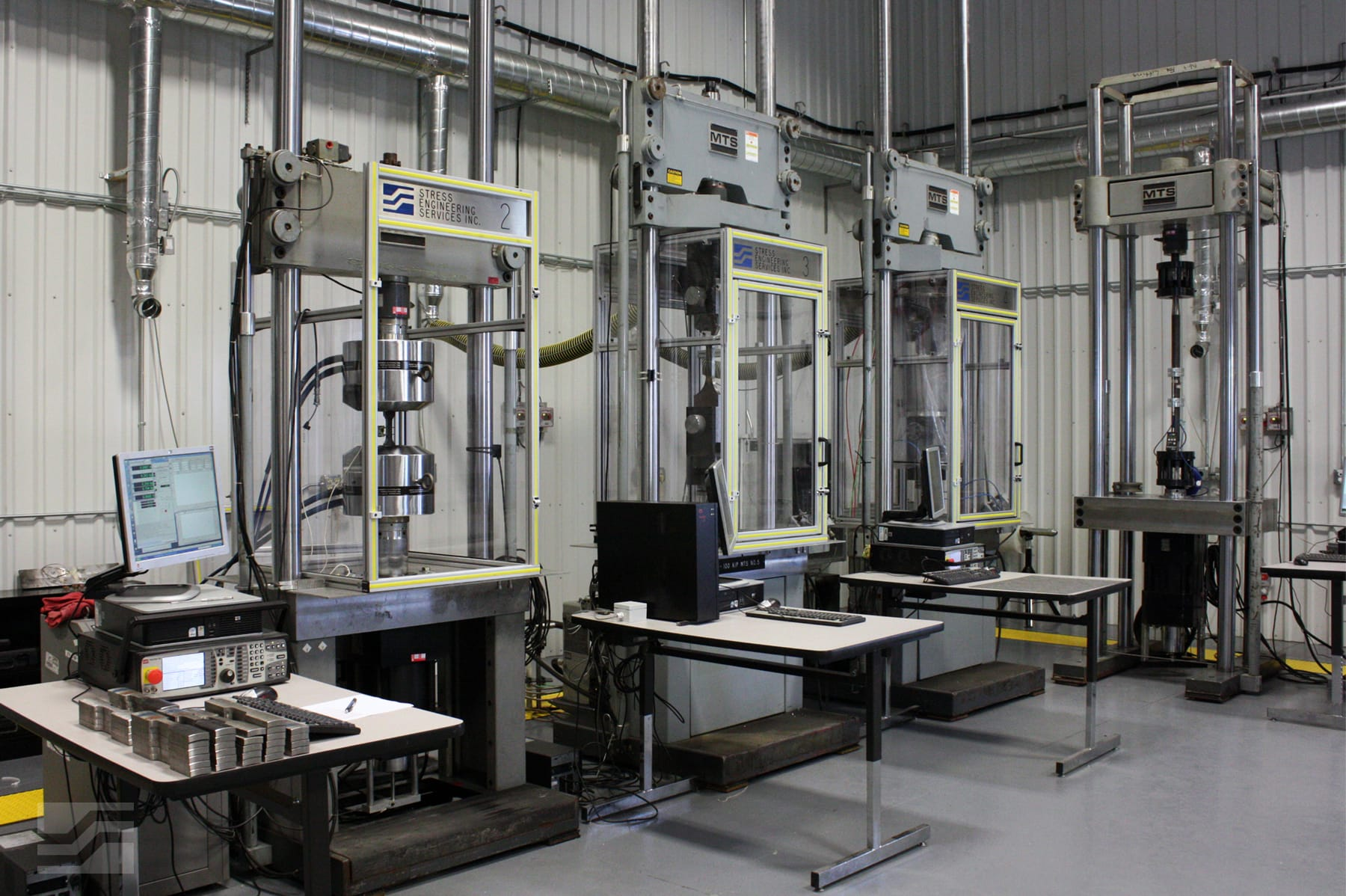### 500,000 Pound Test Frame

• Max Tension/Compression Capacity = 500,000 lbs
• Max Stroke = 6 inches
• Max Sample Length = 8 feet

### 110,000 Pound Test Frame

• Max Tension/Compression Capacity = 110,000 lbs
• Max Stroke = 6 inches
• Max Sample Length = 8 feet

### 55,000 Pound Test Frame

• Max Tension/Compression Capacity = 55,000 lbs
• Max Stroke = 6 inches
• Max Sample Length = 8 feet

### 22,000 Pound Test Frame

• Max Tension/Compression Capacity = 22,000 lbs
• Max Stroke = 6 inches
• Max Sample Length = 2 feet

## PressurePressure Vessels and Chambers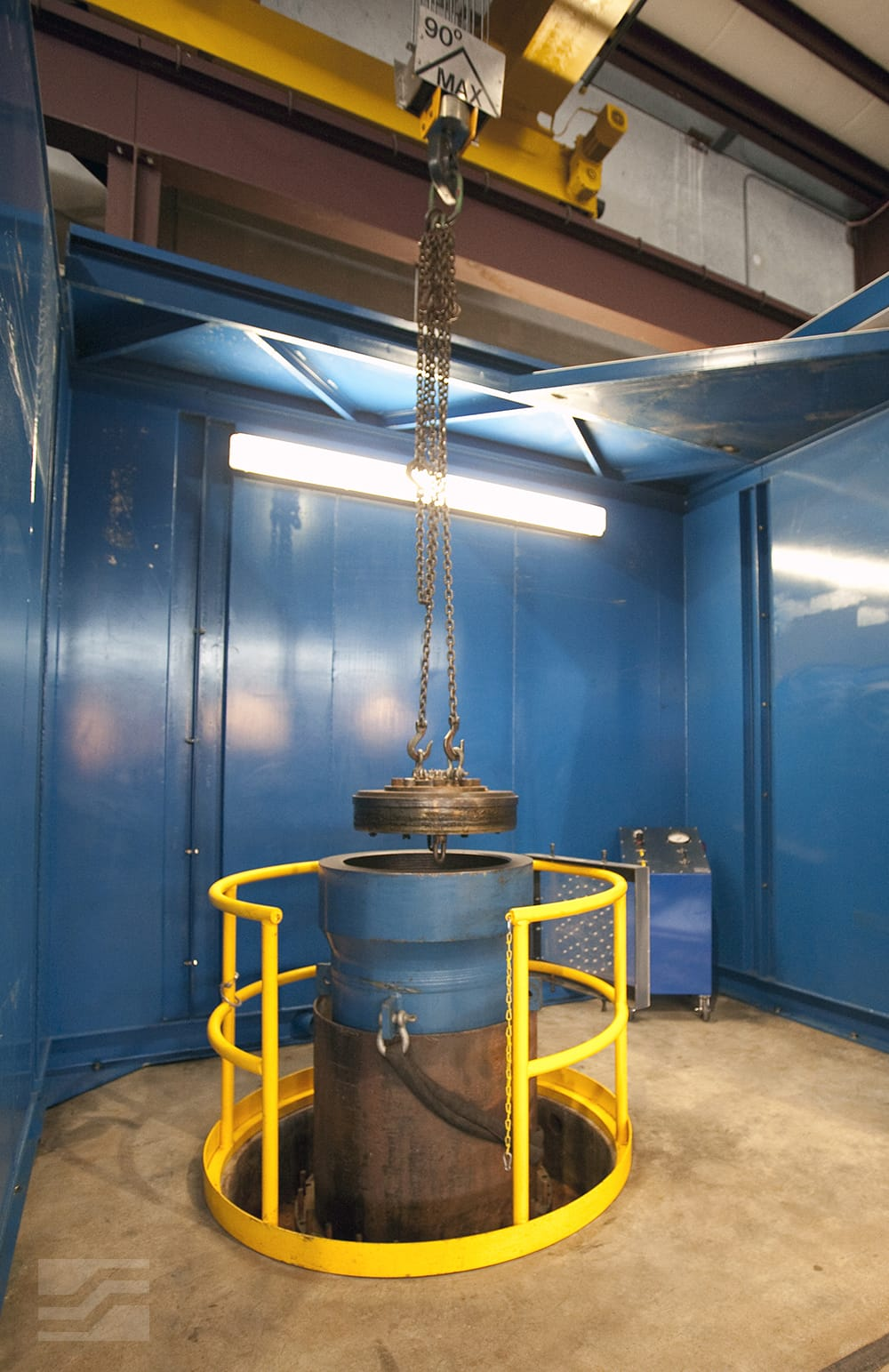### Pressure Vessels and Chambers

 Vessel Max Pressure (psi) Max Inside Diameter (in) Max Sample Length (ft) 1 30,000 * 4.5 15.5 2 30,000 * 5 15.5 3 30,000 * 10 14 4 30,000 * 10 10 5 20,000 6 1.5 6 20,000 5.75 21 7 20,000 4 12 8 20,000 4 3 9 15,000 11 6.5 10 15,000 5.75 24 11 6,000 14 4 12 5,900 42 22 13 4,750 26 11 14 3,700 22 20Slip-On Pressure Chambers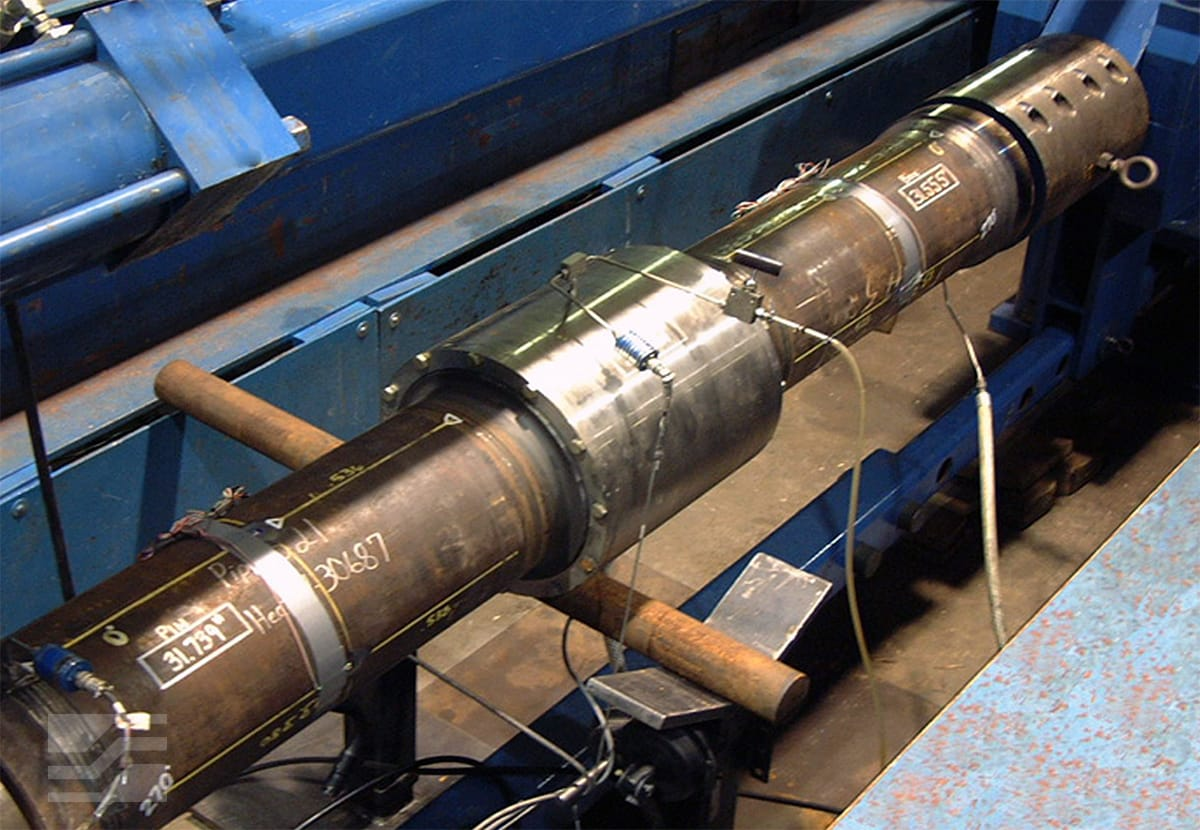### Slip-On Pressure Chambers

• Max Working Pressure = 20,000 psi
• Min Sample Diameter = 2.375 inches O.D.
• Max Sample Diameter = 16 inches O.D.
• Max Sample Length = 6 feet

## FatigueResonant Bending Fatigue Testing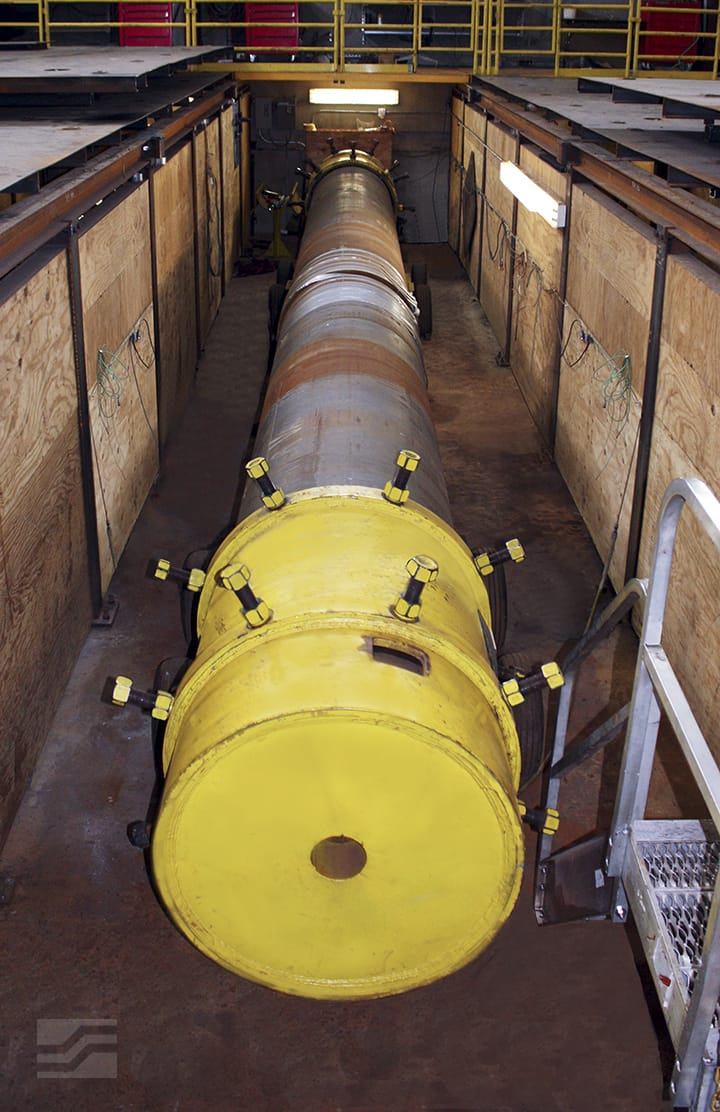### Resonant Bending Fatigue Testing

• Seven machines for 3” to 24“ diameter samples; one machine for 20” to 36“ diameter samples; eight machines for 0.59” to 3“ diameter samples
• Currently have tested from 0.59” to 36” diameter pipes
• Stress ranges from very low to very high possible (typically 5 to 50 ksi range [35 to 350 MPa])
• Cyclic rates typically about 2.5 million cycles per day
• 24 hour / 7 day operation with automatic shutdowns if sample leaks, strain limit is exceeded, or pressure is lost
• Alternating fatigue stresses monitored continuously throughout test
• Post mortem dimensional and metallurgical evaluations to determine misalignment at crack and crack geometryDrill String Friction and Casing/Riser Wear Testing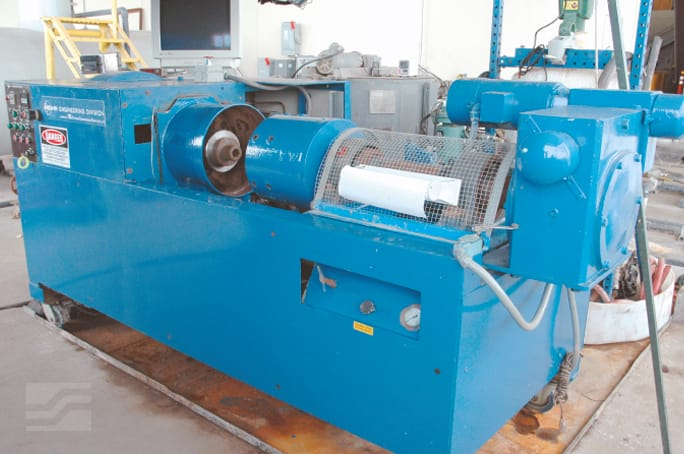### Drill String Friction and Casing/Riser Wear Testing

• Casing = 7 in. to 11.875 in. (plus coupons of larger casing / riser sizes)
• Lateral Load = 10,000 ft-lbs
• Rotary Speed = 155 RPM
• Tool Joint Material = Steel / Hard Metal / Composites
• Tool Joint Size = 3.75 in. (length) / 4.5 in. to 7 in. (diameter)
• Drilling Fluid = Water or Synthetic Based Mud / Brine
• Test Duration = 8 Hours or Until 100% Worn
• Reciprocation Rate = 22 ft./hr. (approx.)